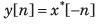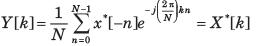Courses

# Test: Fourier Part - 2

## 10 Questions MCQ Test Signal and System | Test: Fourier Part - 2

Description
This mock test of Test: Fourier Part - 2 for Electrical Engineering (EE) helps you for every Electrical Engineering (EE) entrance exam. This contains 10 Multiple Choice Questions for Electrical Engineering (EE) Test: Fourier Part - 2 (mcq) to study with solutions a complete question bank. The solved questions answers in this Test: Fourier Part - 2 quiz give you a good mix of easy questions and tough questions. Electrical Engineering (EE) students definitely take this Test: Fourier Part - 2 exercise for a better result in the exam. You can find other Test: Fourier Part - 2 extra questions, long questions & short questions for Electrical Engineering (EE) on EduRev as well by searching above.
QUESTION: 1

### Consider a continuous time periodic signal x(t) with fundamental period T and Fourier series coefficients X [k]. Determine the Fourier series coefficient of the signal y(t) given in question and choose correct option. Q. y(t) = x(t-t0) + x ( t-t0)

Solution:

x(t- t0) is also periodic with T. The Fourier series coefficients X1 [k] of x(t - t0) are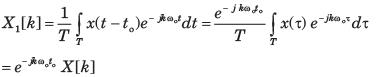Similarly, the FS coefficients of x(t+ t0) are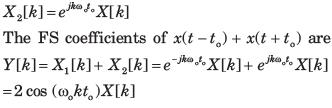QUESTION: 2

### Consider a continuous time periodic signal x(t) with fundamental period T and Fourier series coefficients X [k]. Determine the Fourier series coefficient of the signal y(t) given in question and choose correct option. Q. y(t) = Ev{ x(t)}

Solution: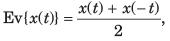The FS coefficients of x(t) are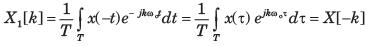Therefore, the FS coefficients of Ev{ x(t)} are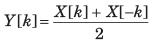QUESTION: 3

### Consider a continuous time periodic signal x(t) with fundamental period T and Fourier series coefficients X [k]. Determine the Fourier series coefficient of the signal y(t) given in question and choose correct option. Q. y(t) = Re{ x(t)}

Solution: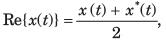The FS coefficient of x *(t) is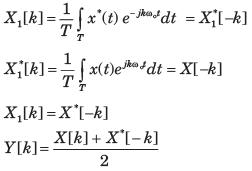QUESTION: 4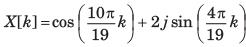Solution: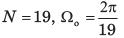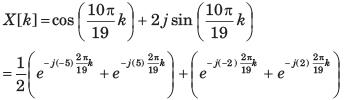By inspection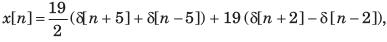Where |n|≤ 9

QUESTION: 5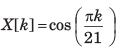Solution: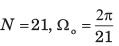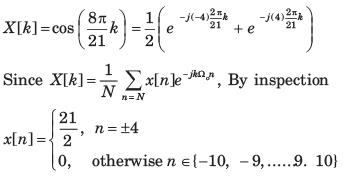QUESTION: 6

Consider a periodic signal x[n] with period N and FS coefficients X [k]. Determine the FS coefficients Y [k] of the signal y[n] given in question.
Q. y[n] = x[n - n0]

Solution: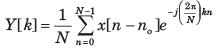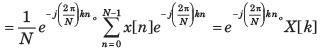QUESTION: 7

Consider a periodic signal x[n] with period N and FS coefficients X [k]. Determine the FS coefficients Y [k] of the signal y[n] given in question.
Q. y[n] = x[n]- x[n - 2 ]

Solution: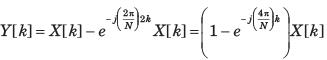QUESTION: 8

Consider a periodic signal x[n] with period N and FS coefficients X [k]. Determine the FS coefficients Y [k] of the signal y[n] given in question.
Q. y[n] = x[n]+ x[n + N 2 ] , (assume that N is even)

Solution: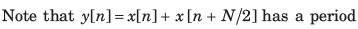of N/2 and N has been assumed to be even,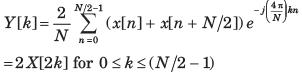QUESTION: 9

Consider a periodic signal x[n] with period N and FS coefficients X [k]. Determine the FS coefficients Y [k] of the signal y[n] given in question.
Q. y[n] = x[n]-x[n-N/2] , (assume that N is even)

Solution:

y[n] = x[n] -x [ n-N/2 ]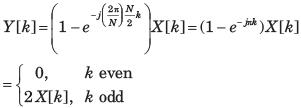QUESTION: 10

Consider a periodic signal x[n] with period N and FS coefficients X [k]. Determine the FS coefficients Y [k] of the signal y[n] given in question.
Q. y[n] = x* [-n]

Solution: